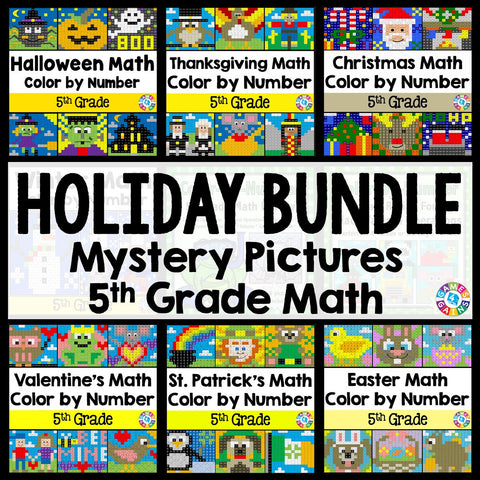## 5th Grade Math Color-by-Number Holiday Bundle

• \$3400
• Save \$8.45

This 5th Grade Math Color-by-Number Holiday Bundle comes to you after receiving numerous requests from buyers to create a bundle that includes all of my seasonal 5th grade math color-by-number sets!

This HUGE bundle covers a wide variety of 5th grade math skills and includes 52 mystery pictures for celebrating all of the major holidays during the school year. Students will have a blast each holiday as they practice key math skills in a fun and COLORFUL way!

Plus, you will SAVE \$\$\$ when you purchase this bundle. If you bought each holiday set individually, you would spend \$40.

Want to learn more about what's included in this bundle? Click on the links below to view the individual previews for each activity. Remember, you'll get all of these holiday sets when you purchase this bundle!

"School Days" Place Value and Operations Color-by-Number Activities (4th/5th Grade)

*Note: This set is a REVIEW of 4th grade math skills, so that 5th grade teachers can use these to review with their incoming 5th graders.

• Writing multi-digit whole numbers using base-ten numerals, number names, and expanded form
• Writing fractions with denominators 10 or 100 as decimals
• Round multi-digit whole numbers
• Identifying the factors of a number within 100
• Multiplying larger numbers
• Dividing larger numbers

Halloween Math Color-by-Number Activities (5th Grade)

• Writing numbers with exponents in standard form
• Writing decimals
• Rounding decimals
• Comparing decimals
• Adding and subtracting decimals using the standard algorithm
• Adding and subtracting decimals in one-step and two-step word problems

Thanksgiving Math Color-by-Number Activities (5th Grade)

• Multiplying and dividing decimals by 10, 100, and 1,000 (with the decimals in the problem written in standard, word, and expanded form)
• Ordering decimals
• Subtracting decimals from whole numbers
• Multiplying whole numbers (up to 3-digit x 3-digit and 4-digit x 2-digit)
• Solving multi-step word problems (whole numbers with addition, subtraction, and multiplication)
• Evaluating expressions with parentheses (whole numbers with addition, subtraction, and multiplication)

Christmas Math Color By Number (5th Grade)

• Evaluating expressions
• Reading and writing decimals in different forms
• Dividing whole numbers (up to 4-digit by 2-digit, no remainders)
• Multiplying decimals by whole numbers (decimal to hundredths by up to a 3-digit whole number)
• Adding and subtracting proper fractions with unlike denominators
• Solving area, perimeter, and volume problems

Winter Math Color By Number (5th Grade)

• Reading and writing decimals word problems
• Rounding and comparing decimals word problems
• Adding and subtracting decimals word problems
• Multiplying and dividing decimals word problems

Valentine's Day Math Color-by-Number Activities (5th Grade)

• Multiplying decimals to hundredths
• Dividing decimals to hundredths by single-digit and double-digit numbers
• Adding proper fractions with unlike denominators with answers expressed as a mixed number
• Converting metric measurements
• Determining the volume of rectangular prisms made from centimeter cubes
• Solving problems from line plots with fractional measurements

St. Patrick's Day Math Color-by-Number Activities (5th Grade)

• Solving word problems involving division of whole numbers land answers in the form of fractions or mixed numbers
• Converting customary measurements with answers in the form of fractions or mixed numbers
• Determining the next number in a pattern (with decimals)
• Determining the volume or missing side length of a rectangular prism
• Classifying two-dimensional shapes
• Identifying points in the first quadrant of a coordinate plane

Easter Math Color-by-Number Activities (5th Grade)

• Dividing decimals by decimals (up to hundredths)
• Multiplying fractions by fractions and whole numbers
• Determining the pattern to solve the missing number in a table
• Plotting points on the coordinate plane to form geometric shapes (types of triangles and quadrilaterals)
• Calculating area with fractional side lengths
• Calculating volume when 2 or more rectangular prisms are combined

Summer Math Color-by-Number Activities (5th Grade)

• Adding, subtracting, multiplying, and dividing fractions
• Adding, subtracting, multiplying, and dividing decimals to hundredths
• Solving expressions with parentheses
• Converting measurements with decimals
• Calculating the volume of rectangular prisms, including additive volume
• Identifying points in the coordinate plane